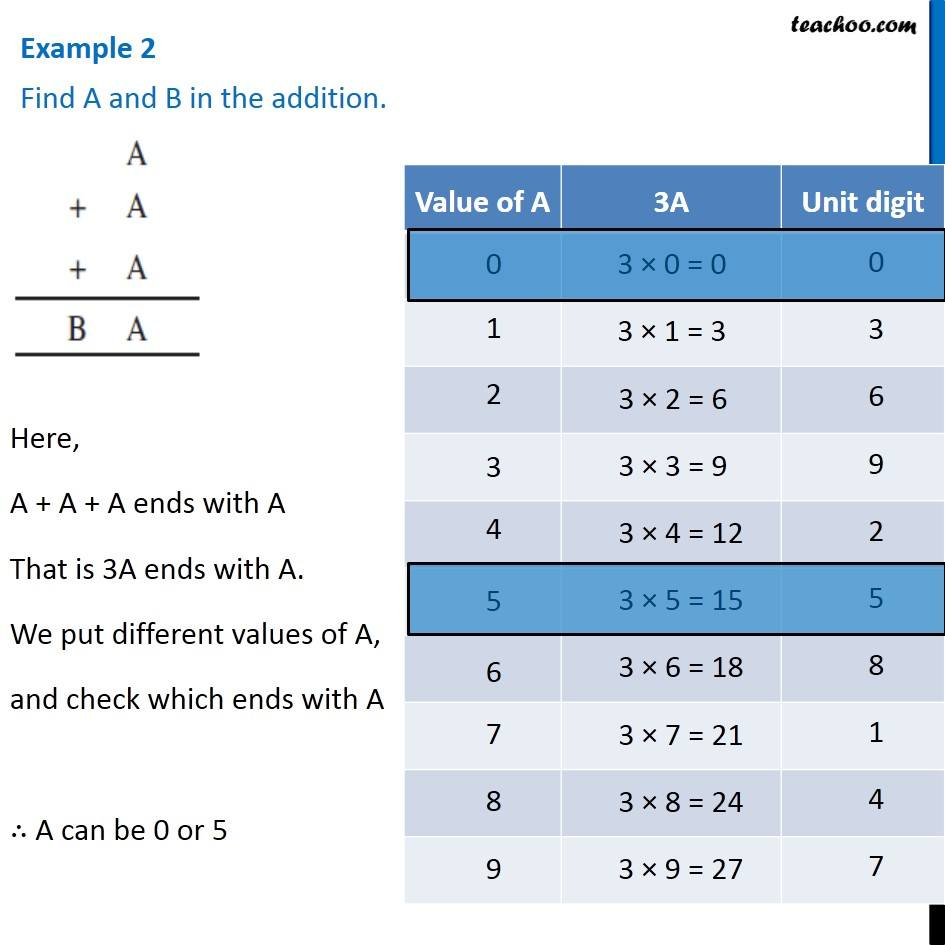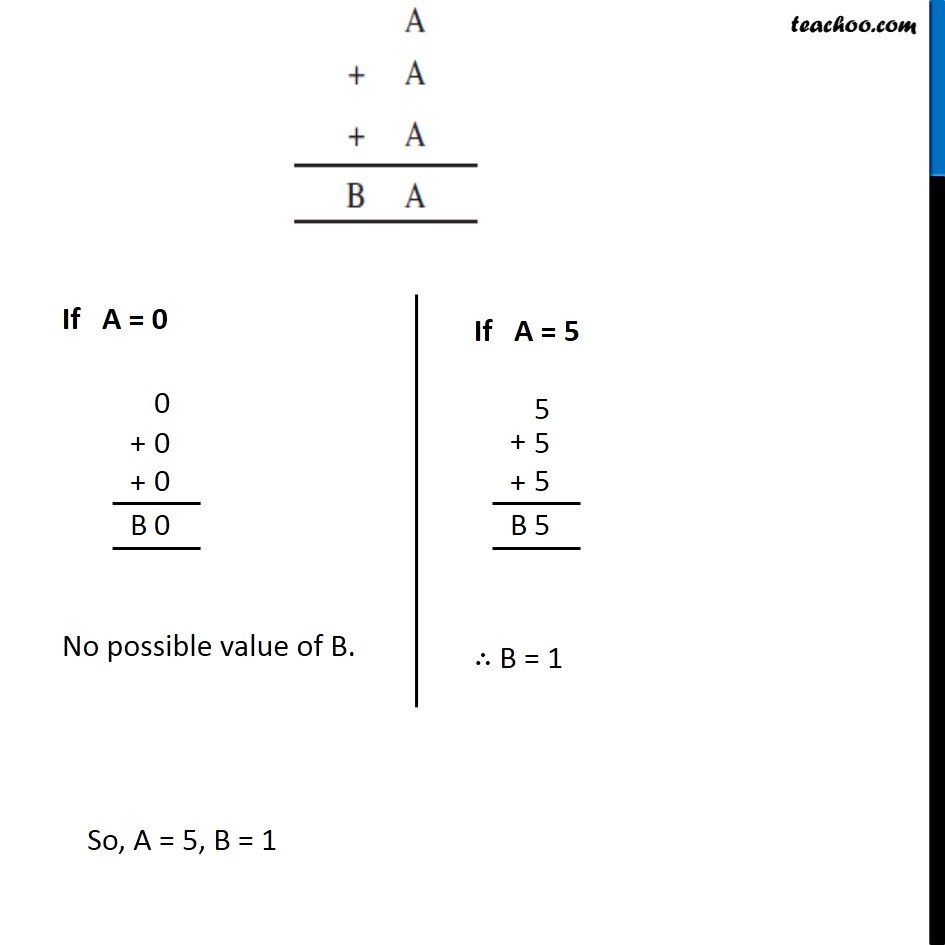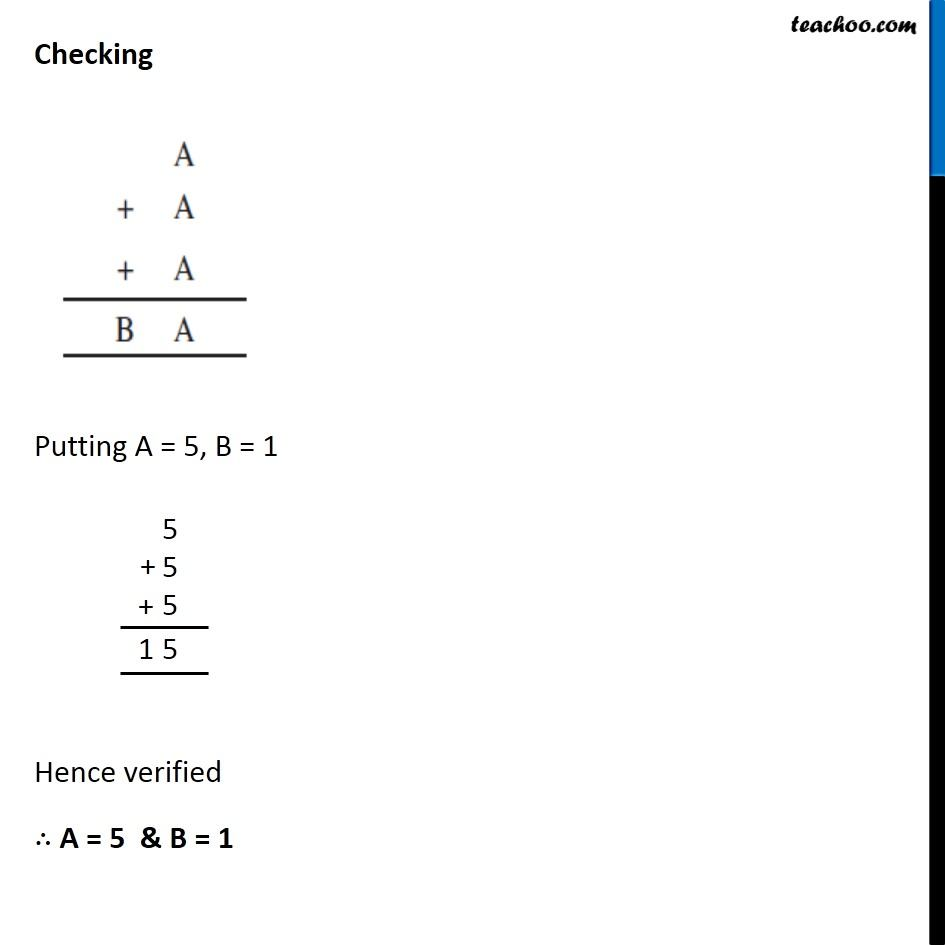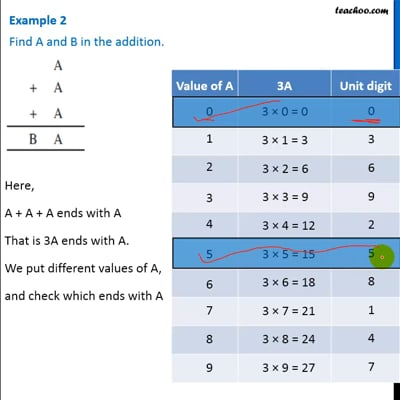Examples

Chapter 16 Class 8 Playing with Numbers
Serial order wiseThis video is only available for Teachoo black users

### Transcript

Example 2 Find A and B in the addition. Here, A + A + A ends with A That is 3A ends with A. We put different values of A, and check which ends with A ∴ A can be 0 or 5 If A = 0 No possible value of B. If A = 5 ∴ B = 1 So, A = 5, B = 1 Checking Putting A = 5, B = 1 Hence verified ∴ A = 5 & B = 1